#### 期刊菜单

Effects of Persistent Sodium and Calcium Current on Mixed Bursting in the Respiratory Neuron with Dynamical Analysis

Abstract: The experimental study found that the pre-Bötzinger complex in the mammalian brain stem is the key part of the generation of respiratory rhythm, which contains many dynamic behaviors. By changing calcium activated nonspecific cation current and persistent sodium current, such as the inspiratory neuron model, a series of special somatic-dendritic mixed bursting patterns can be generated, that is, there are multiple types of bursting patterns in the same cycle. Dynamic methods such as fast-slow decomposition and bifurcation analysis were used to explore the generation and transition mechanism of somatic-dendritic mixed bursting, and it was found that the changes of calcium activated nonspecific cation current and persistent sodium current lead to changes in the number and type of mixed cluster discharge, providing theoretical support for future research on the origin of respiratory movement rhythm.

1. 引言

1991年，Butera等人为pre-BötC中的单个和耦合神经元细胞分别建立了基于持续钠电流( ${I}_{NaP}$ )的数学模型   。Toporikova和Butera提出了一个pre-Bötzinger神经元中的双腔室电导模型(TB模型)，结果表明此模型可以产生依赖于持续钠电流( ${I}_{NaP}$ )和钙激活非特异性阳离子电流( ${I}_{CAN}$ )的神经元放电    。随后，Park和Rubin对TB模型进行了简化处理，提出了一个pre-BötC的单腔室电导模型，此模型可以和双室模型产生同样的放电类型  。Rinzel率先使用快慢变量分离和分岔分析的动力学分析方法，为人们后续理解神经元放电活动的产生机理建立了科学依据     。Dunmyre等人也提出了混合放电的产生机制的新解释方法  。此外，Wang和Rubin等人还观察到了混合放电(MB)，并且对胞体–树突簇放电(S-D Bursting)的参数区域做出了分类  。Duan等人研究了耦合神经元的同相簇放电和反向簇放电的放电模式，并对其进行了分类   。Wang等人研究了耦合pre-BötC模型中混合放电的动力学机制  。随着研究的深入，Lü和Chen等人在单个pre-Bötzinger神经元中发现了大量混合放电模式，对其产生进行动力学分析并将其分类   。这些研究成果为我们研究呼吸系统神经元混合簇放电提供了重要帮助，但影响胞体–树突簇放电的因素还有很多，需要我们逐步完善。

2. 模型介绍

$\frac{\text{d}V}{\text{d}t}=\left(-{I}_{NaP}-{I}_{Na}-{I}_{K}-{I}_{L}-{I}_{CAN}\right)/C$ (3-1)

$\frac{\text{d}n}{\text{d}t}=\left({n}_{\infty }\left(V\right)-n\right)/{\tau }_{n}\left(V\right)$ (3-2)

$\frac{\text{d}h}{\text{d}t}=\left({h}_{\infty }\left(V\right)-h\right)/{\tau }_{h}\left(V\right)$ (3-3)

${I}_{Na}={g}_{Na}{m}_{\infty }^{3}\left(V\right)\left(1-n\right)\left(V-{V}_{Na}\right)$

${I}_{NaP}={g}_{NaP}m{p}_{\infty }\left(V\right)h\left(V-{V}_{Na}\right)$

${I}_{L}={g}_{L}\left(V-{V}_{L}\right)$

${I}_{K}={g}_{K}{n}^{4}\left(V-{V}_{K}\right)$

${I}_{CAN}={g}_{CAN}f\left(\left[Ca\right]\right)\left(V-{V}_{Na}\right)$

$m{p}_{\infty }\left(V\right)=1/\left(1+\mathrm{exp}\left(\left(V-{\theta }_{mp}\right)/{\sigma }_{mp}\right)\right)$

${m}_{\infty }\left(V\right)=1/\left(1+\mathrm{exp}\left(\left(V-{\theta }_{m}\right)/{\sigma }_{m}\right)\right)$

${n}_{\infty }\left(V\right)=1/\left(1+\mathrm{exp}\left(\left(V-{\theta }_{n}\right)/{\sigma }_{n}\right)\right)$

${h}_{\infty }\left(V\right)=1/\left(1+\mathrm{exp}\left(\left(V-{\theta }_{h}\right)/{\sigma }_{h}\right)\right)$

${\tau }_{n}\left(V\right)={\tau }_{n}/\mathrm{cosh}\left[\left(V-{\theta }_{n}\right)/\left(2{\sigma }_{n}\right)\right]$

${\tau }_{h}\left(V\right)={\tau }_{h}/\mathrm{cosh}\left[\left(V-{\theta }_{h}\right)/\left(2{\sigma }_{h}\right)\right]$

$f\left(\left[Ca\right]\right)=1/\left(1+{\left({k}_{CAN}/\left[Ca\right]\right)}^{{n}_{CAN}}\right)$

$\frac{\text{d}\left[Ca\right]}{\text{d}t}={f}_{i}\left({J}_{E{R}_{IN}}-{J}_{E{R}_{OUT}}\right)$ (3-4)

$\frac{\text{d}l}{\text{d}t}=A{K}_{d}\left(1-l\right)-A\left[Ca\right]l$ (3-5)

${J}_{E{R}_{IN}}=\left({L}_{I{P}_{3}}+{P}_{I{P}_{3}}{\left[\frac{\left[I{P}_{3}\right]\left[Ca\right]l}{\left(\left[I{P}_{3}\right]+{K}_{I}\right)\left(\left[Ca\right]+{K}_{a}\right)}\right]}^{3}\right)×\left(\frac{{\left[Ca\right]}_{Tot}-\left[Ca\right]}{\sigma }-\left[Ca\right]\right)$

${J}_{E{R}_{OUT}}={V}_{SERCA}\frac{{\left[Ca\right]}^{2}}{{K}_{SERCA}+{\left[Ca\right]}^{2}}$ (3-6)

3. 钙激活非特异性阳离子电流对胞体–树突混合簇放电的影响

3.1. ${k}_{CAN}$ 变化对胞体–树突混合簇放电的影响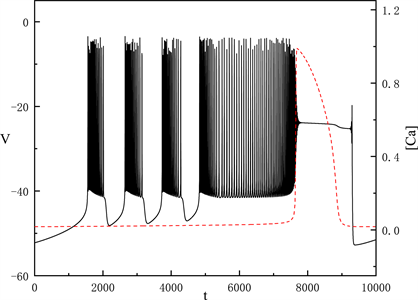Figure 1. Time series of the membrane potential V with ${k}_{CAN}=0.12\text{\hspace{0.17em}}\mu \text{M}$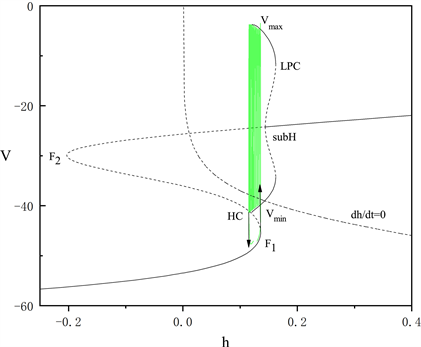Figure 2. The fast-slow decomposition and bifurcation analysis of the first bursting in the whole system with ${k}_{CAN}=0.12\text{\hspace{0.17em}}\mu \text{M}$ and $\left[Ca\right]=0.019$ fixed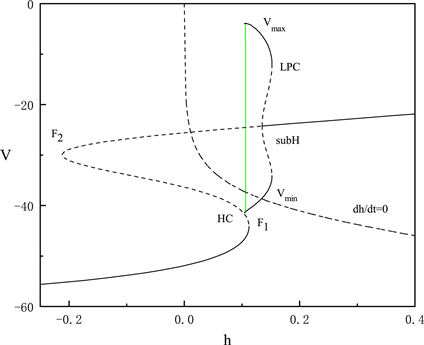Figure 3. The fast-slow decomposition and bifurcation analysis of the last spiking in the whole system with ${k}_{CAN}=0.12\text{\hspace{0.17em}}\mu \text{M}$ and $\left[Ca\right]=0.028$ fixed

$\left[Ca\right]$ 固定为0.028时，对胞体后段的放电模式进行了快慢变量分离和分岔分析。图3中显示胞体后段的放电类型为峰放电。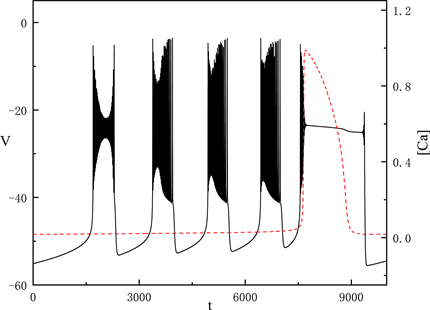Figure 4. Time series of the membrane potential V with ${k}_{CAN}=0.45\text{\hspace{0.17em}}\mu \text{M}$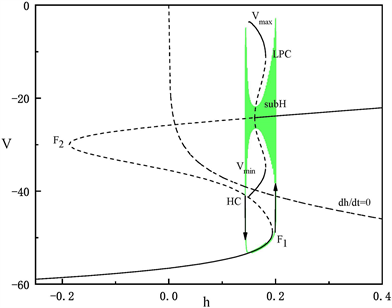Figure 5. The fast-slow decomposition and bifurcation analysis of the first bursting in the whole system with ${k}_{CAN}=0.45\text{\hspace{0.17em}}\mu \text{M}$ and $\left[Ca\right]=0.019$ fixed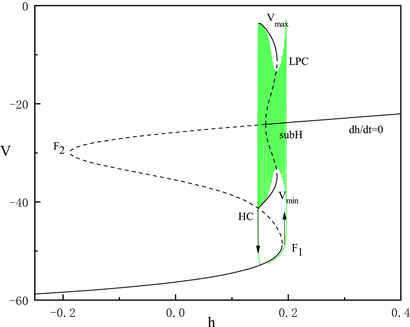Figure 6. The fast-slow decomposition and bifurcation analysis of the second bursting in the whole system with ${k}_{CAN}=0.45\text{\hspace{0.17em}}\mu \text{M}$ and $\left[Ca\right]=0.022$ fixed

3.2. ${g}_{CAN}$ 变化对胞体–树突混合簇放电的影响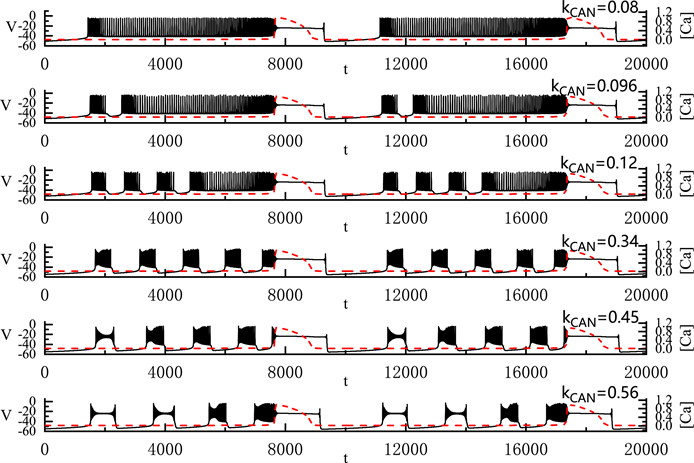Figure 7. Variation of the somatic-dendritic mixed bursting with ${k}_{CAN}=0.08,0.096,0.12,0.34,0.45$ and 0.56 μM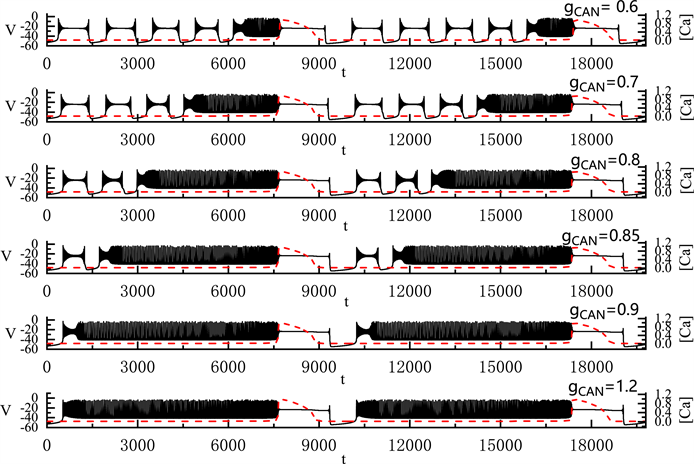Figure 8. Variation of the somatic-dendritic mixed bursting with ${g}_{CAN}=0.6,0.7,0.8,0.85,0.9$ and 1.2 nS

4. 持续钠离子电流对胞体–树突混合簇放电的影响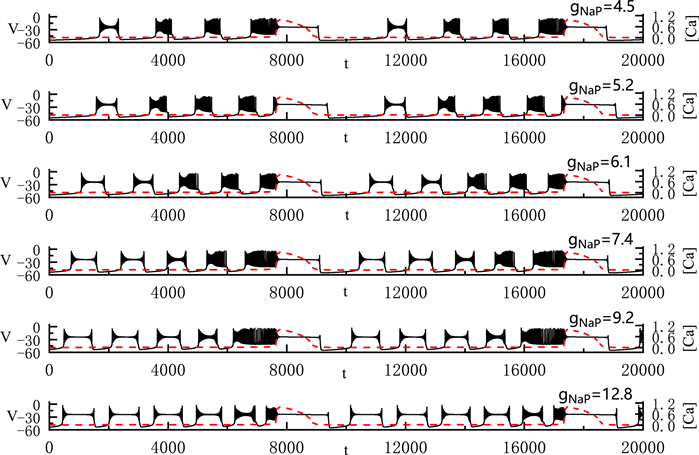Figure 9. Variation of the somatic-dendritic mixed bursting with ${g}_{NaP}=4.5,5.2,6.1,7.4,9.2$ and 12.8 nS

5. 结语

  Smith, J.C., Ellenberger, H.H., Ballanyi, K. and Feldman, J.L. (1991) Pre-Bötzinger Complex: A Brainstem Region that May Generate Respiratory Rhythm in Mammals. Science, 254, 726-729. https://doi.org/10.1126/science.1683005  Butera, R.J., Rinzel, J. and Smith, J.C. (1999) Models of Respiratory Rhythm Generation in the Pre-Bötzinger Complex. I. Bursting Pacemaker Neurons. Journal of Neurophysiology, 82, 382-397. https://doi.org/10.1152/jn.1999.82.1.382  Butera, R.J., Rinzel, J. and Smith, J.C. (1999) Models of Respiratory Rhythm Generation in the Pre-Bötzinger Complex. II. Populations of Coupled Pacemaker Neurons. Journal of Neurophysiology, 82, 398-415. https://doi.org/10.1152/jn.1999.82.1.398  Paton, J., Abdala, A., Koizumu, H., Smith, J.C. and St-John, W.M. (2006) Respiratory Rhythm Generation during Gasping Depends on Persistent Sodium Current. Nature Neuroscience, 9, 311-313. https://doi.org/10.1038/nn1650  Pace, R.W., Machay, D.D., Feldman, J.L. and Del Negro, C.A. (2007) Inspiratory Bursts in the Pre-Bötzinger Complex Depend on a Calcium-Activated Non-Specific Cation Current Linked to Glutamate Receptors in Neonatal Mice. The Journal of Physiology, 582, 113-125. https://doi.org/10.1113/jphysiol.2007.133660  Toporikova, N. and Butera, R.J. (2011) Two Types of Independent Bursting Mechanisms in Inspiratory Neurons: An Integrative Model. Journal of Computational Neuroscience, 30, 515-528. https://doi.org/10.1007/s10827-010-0274-z  Park, C. and Rubin, J.E. (2013) Cooperation of Intrinsic Bursting and Calcium Oscillations Underlying Activity Patterns of Model Pre-Bötzinger Complex Neurons. Journal of Computational Neuroscience, 34, 345-366. https://doi.org/10.1007/s10827-012-0425-5  Rinzel, J. (1986) Excitation Dynamics: Insights from Simplified membrane Models. Federation Proceedings, 44, 2944-2946.  Gu, H.G., Pan, B.B., Chen, G.R. and Duan, L.X. (2014) Biological Experimental Demonstration of Bifurcations from Bursting to Spiking Predicted by Theoretical Models. Nonlinear Dynamics, 78, 391-407. https://doi.org/10.1007/s11071-014-1447-5  Lü, Z.S., Zhao, C., Zhang, B.Z. and Duan, L.X. (2017) Multitime Scale Study of Bursting Activities in the Pre- Bötzinger Complex. International Journal of Bifurcation & Chaos, 27, Article ID: 1750172. https://doi.org/10.1142/S0218127417501723  Zhan, F.B., Liu, S.Q. and Zhang, X.H. (2018) Mixed-Mode Oscillations and Bifurcation Analysis in a Pituitary Model. Nonlinear Dynamics, 94, 807-826. https://doi.org/10.1007/s11071-018-4395-7  Dunmyre, J.R., Del Negro, C.A. and Rubin, J.E. (2011) Interactions of Persistent Sodium and Calcium-Activated Nonspecific Cationic Currents Yield Dynamically Distinct Bursting Regimes in a Model of Respiratory Neurons. Journal of Computational Neuroscience, 31, 305-328. https://doi.org/10.1007/s10827-010-0311-y  Wang, Y.Y. and Rubin, J.E. (2016) Multiple Timescale Mixed Bursting Dynamics in a Respiratory Neuron Model. Journal of Computational Neuroscience, 41, 245-268. https://doi.org/10.1007/s10827-016-0616-6  Duan, L.X., Liu, J., Chen, X., Xiao, P.C. and Zhao, Y. (2016) Dynamics of In-Phase and Anti-Phase Bursting in the Coupled Pre-Bötzinger Complex Cells. Cognitive Neurodynamics, 11, 91-97. https://doi.org/10.1007/s11571-016-9411-3  Zhao, Y.Q., Liu, M.T., Zhao, Y. and Duan, L.X. (2021) Dynamics of Mixed Bursting in Coupled Pre-Bötzinger Complex. Acta Physica Sinica, 70, Article ID: 120501. https://doi.org/10.7498/aps.70.20210093  Wang, Z.J., Duan, L.X. and Cao, Q.Y. (2018) Multi-Stability Involved Mixed Bursting within the Coupled Pre- Bötzinger Complex Neurons. Chinese Physics B, 27, Article ID: 070502. https://doi.org/10.1088/1674-1056/27/7/070502  Lü, Z.S., Liu, M.R. and Duan, L.X. (2019) Bifurcation Analysis of Mixed Bursting in the Pre-Bötzinger Complex. Applied Mathematical Modelling, 67, 234-251. https://doi.org/10.1016/j.apm.2018.10.031  Lü, Z.S., Liu, M.R. and Duan, L.X. (2021) Dynamical Analysis of Dendritic Mixed Bursting within the Pre-Bötzinger Complex. Nonlinear Dynamics, 103, 897-912. https://doi.org/10.1007/s11071-020-06097-1  Viemari, J. and Ramirez, J. (2006) Norepinephrine Differentially Modulates Different Types Of Respiratory Pacemaker and Nonpacemaker Neurons. Journal of Neurophysiology, 95, 2070-2082. https://doi.org/10.1152/jn.01308.2005  Atsushi, D., Sebastien, Z., Frank, E., et al. (2011) Upregulation of Norepinephrine (NE) Causes Instability of Respiratory Rhythm under Acute Intermitted Hypoxia (AIH) at in Vivo Whole Mice. The FASEB Journal, 25, 1074.2. https://doi.org/10.1096/fasebj.25.1_supplement.1074.2  Tang, Q., Ma, J.H., Zhang, P.H., Wan, W., Kong, L.H. and Wu, L. (2012) Persistent Sodium Current and Na+/H+ Exchange Contributes to the Augmentation of the Reverse Na+/Ca2+ Exchange during Hypoxia or acute Ischemia in Ventricular Myocytes. Pflügers Archiv-European Journal of Physiology, 463, 513-522. https://doi.org/10.1007/s00424-011-1070-y  Mantegazza, M., Franceschetti, S. and Avanzini, G. (1998) Anemone Toxin (ATX II)-Induced Increase in Persistent Sodium Current: Effects on the Firing Properties of Rat Neocortical Pyramidal Neurons. The Journal of Physiology, 507, 105-116. https://doi.org/10.1111/j.1469-7793.1998.105bu.x  Wengert, E.R. and Patel, M.K. (2020) The Role of the Persistent Sodium Current in Epilepsy. Epilepsy Currents, 21, 40-47. https://doi.org/10.1177/1535759720973978